# Excel VLOOKUP with Dynamic Column Reference

• Last Updated : 24 Jul, 2022

Suppose anytime you work with colossal tables of data and you want to implant a VLOOKUP function that dynamically updates to the accompanying portion as you copy it across. In that case, the VLOOKUP with the COLUMNS capacity is what you truly care about. That is; the col_index_num a piece of the VLOOKUP capacity intensely revives as you copy it across your worksheet. Before learning VLOOKUP with Dynamic Column Reference, let’s first learn what is VLOOKUP function,

## VLOOKUP

VLOOKUP is utilized when one necessities to track down the worth of a cell/data of that cell that is available elsewhere (can likewise be available in another sheet). The following is the capability of VLOOKUP,

=VLOOKUP(lookup_value,table_array,col_index_num,[range_lookup])

Example: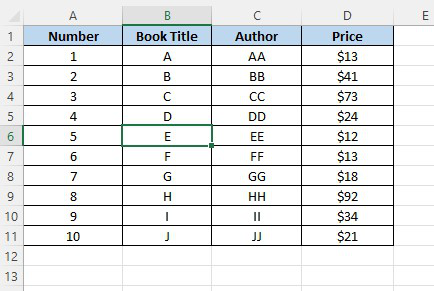Okay, we ought to imagine this summary is hundreds or even a considerable number of sections long and we really want to find a quick strategy for getting expenses and maker information on demand (other than CTRL+F for Find clearly). In the table underneath we have the Book Title, Author, and Price.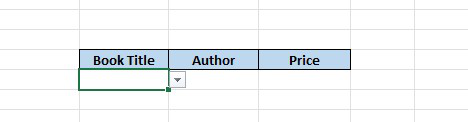The book title is found utilizing a Data Validation List or Drop Down List, and in sections C and D we want a VLOOKUP equation that finds the Author and Price separately. We could do this utilizing a standard VLOOKUP recipe like this: In cell C16,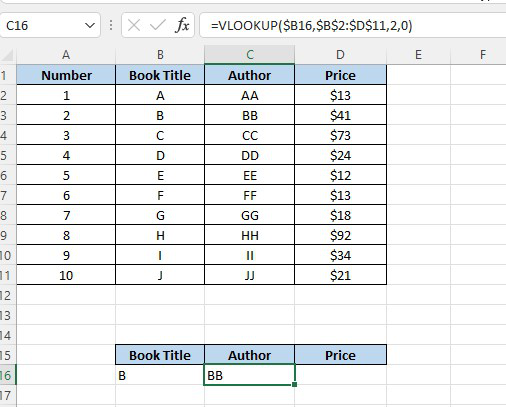What’s more, in cell D16,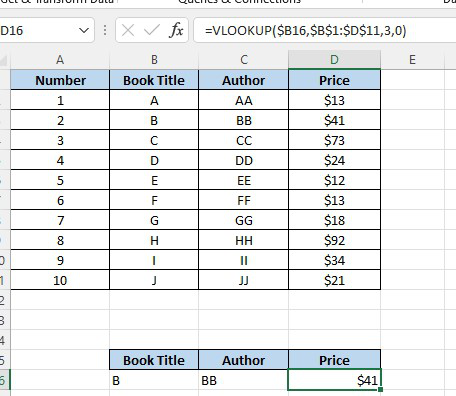Yet, we want to physically alter the section reference for every segment we duplicate the VLOOKUP across. Furthermore, assuming we have a ton of segments that we need to look into, then, at that point, rather than hard coding the section reference number, it’s much faster to utilize the COLUMNS capability like this: In cell C16,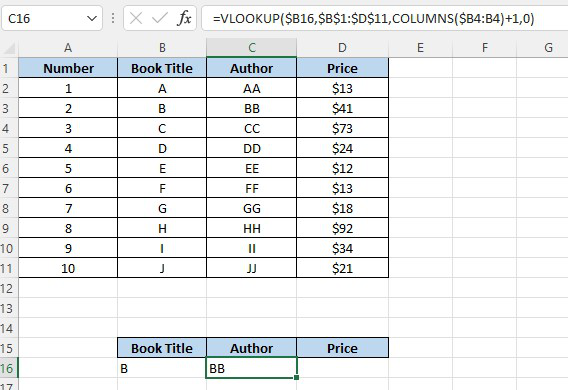What’s more, in cell D16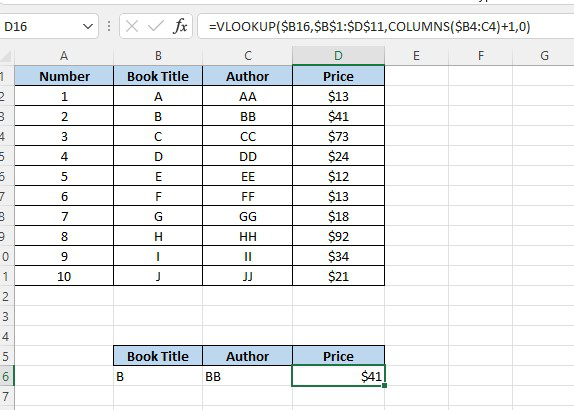Note: Make sure you utilize the COLUMNS (plural) not COLUMN, as they work in an unexpected way.

## COLUMNS function

The COLUMNS capability returns the quantity of sections in a cluster. The language structure is =COLUMNS(array), where ‘cluster’ is the section range. For instance:

=COLUMNS(\$B4:B4)

It gives us 1. For example, the exhibit is 1 section wide. So all we’re truly doing is working out the segment number we need utilizing the COLUMNS capability.

Note: \$B4:B4 could simply been plain B:B like this =COLUMNS(\$B:B), as it doesn’t exactly make any difference what the column reference is.

## VLOOKUP using COLUMNS Function Explained

In our VLOOKUP equation above (in cell C16), our COLUMNS recipe is COLUMNS(\$B4:B4)+1, because,

• The segment we need returned is the second section in our VLOOKUP table,
• What’s more, since =COLUMNS(\$B4:B4) assesses to 1 we really want to add 1 to get 2 for the subsequent segment.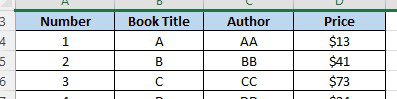In the VLOOKUP function in cell D16 our COLUMNS capability is COLUMNS(\$B4:C4)+1 and it assesses to 2+1=3. We’ve utilized an outright cell reference on \$B4:C4 (=2) so that when we replicated the VLOOKUP recipe opposite C16 to D16, the COLUMNS a piece of the equation will naturally increment by 1 (from \$B4:B4 to \$B4:C4) to give us the right segment number.

## Two-way lookup with VLOOKUP (Match)

Inside the VLOOKUP capability, the section record contention is regularly hard-coded as a static number. Be that as it may, you can likewise make a unique segment file by utilizing the MATCH capability to find the right section. This strategy permits you to make a powerful two-way query, matching on the two lines and sections. It can likewise make a VLOOKUP recipe stronger: VLOOKUP can break when sections are embedded or eliminated from a table, however an equation with VLOOKUP + MATCH can keep on working accurately even changes are made to segments.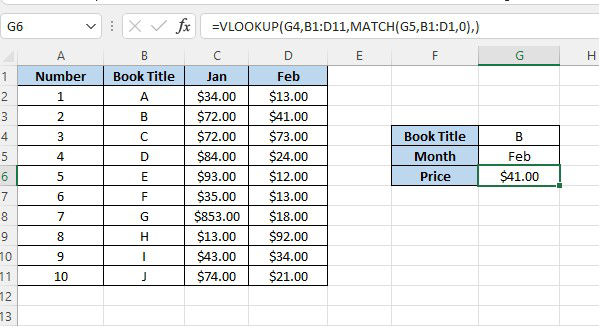In the model, we are utilizing this recipe to progressively query the two lines and sections with VLOOKUP:

=VLOOKUP(G4,B1:D11,MATCH(G5,B1:D1,0),0)

### Explanation

This is a standard VLOOKUP careful coordinate equation with one exemption: the section file is provided by the MATCH capability.

Note that the query cluster given to MATCH (B1:D1) addressing section headers intentionally incorporates the vacant cell B1. This is done so the number returned by MATCH is in a state of harmony with the table utilized by VLOOKUP. As such, you really want to give MATCH a reach that traverses similar number of segments VLOOKUP is involving in the table. In the model (for Feb) MATCH returns 3, so after MATCH runs, the VLOOKUP recipe seems to be this:

=VLOOKUP(G4,B1:D11,3,0)

Which returns deals for B (line 3) in Feb (section 4), which is \$41.

My Personal Notes arrow_drop_up